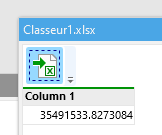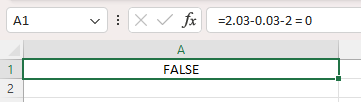# Excel displays 35491533,8273083 and Easymorph displays 35491533,8273084

Hi,

I have an excel file showing the number in one cell : 35491533,8273083.
When I rename the excel file as zip, and then goes to xl>worksheets>sheet1.xml the real number is 35491533,827308349. So everything is fine in Excel because it rounds correctly.

However if I import the same excel file easymorph shows 35491533,8273084 (version 5.4.2)

See example attached
Classeur1.xlsx (9.8 KB)Can you please investigate ? It can cause trouble when you sum many amounts like that => sum can be very different between tools.

Hi Romain,

Numbers in EasyMorph and in Excel are represented differently:

Excel represents numbers as floating-point numbers, which results in subtle errors in calculations. For instance, 2.03 - 0.03 - 2 is not equal to 0 in Excel.EasyMorph numbers are fixed-point decimals and therefore it doesn't have such errors. However, when data is imported from Excel into EasyMorph it's converted from floating-point numbers to fixed-point numbers. Therefore rounding discrepancies like the one you described can occur.

Similar discrepancies may also occur when data is exported from EasyMorph to Excel or any other data source that uses floating-point numbers.

I'm not sure to understand. In the excel file attached the number in the cell is 35491533,827308349, hard-coded precisely in the xml data file of the excel file.

So why in Easymorph would it be rounded 35491533,8273084 ?
Does it mean that when you import an excel file, you are using a kind of Microsoft API that may have trouble to "identify" the good initial number ? Not sure to understand.

We're looking closer at the issue.